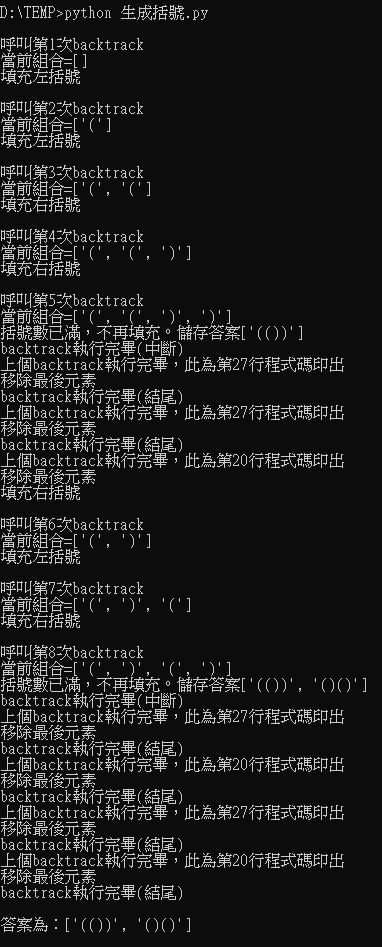0

python backtrack的問題

def generateParenthesis(self, n):
ans = []
def backtrack(S, left, right):
if len(S) == 2 * n:
ans.append(''.join(S))
return
if left < n:
S.append('(')
backtrack(S, left+1, right)
S.pop()

if right < left:
S.append(')')
backtrack(S, left, right+1)
S.pop()

#本人非本科，自學菜雞求救

2 個回答

0

def backtrack(當前組合, 左括號數, 右括號數):
global 次數
次數 += 1
print('\n呼叫第' + str(次數) + '次backtrack')
print('當前組合=' + str(當前組合))

if len(當前組合) == 2 * 括號對數:
答案.append(''.join(當前組合))
print('括號數已滿，不再填充。儲存答案' + str(答案))
print('backtrack執行完畢(中斷)')
return
if 左括號數 < 括號對數:
當前組合.append('(')
print('填充左括號')
backtrack(當前組合, 左括號數+1, 右括號數)
print('上個backtrack執行完畢，此為第20行程式碼印出')
當前組合.pop()
print('移除最後元素')
if 右括號數 < 左括號數:
當前組合.append(')')
print('填充右括號')
backtrack(當前組合, 左括號數, 右括號數+1)
print('上個backtrack執行完畢，此為第27行程式碼印出')
當前組合.pop()
print('移除最後元素')
print('backtrack執行完畢(結尾)')

backtrack([], 0, 0)
print('\n答案為：' + str(答案))"上個Backtrack執行完畢，此為第20行程式碼印出"
"移除最後元素"
"填充右括號" <<<<<<(???)

uobik iT邦新手 4 級 ‧ 2021-06-30 18:56:46 檢舉

"就繼續往後執行，遇到第三個if判斷，有符合(右括號數 < 左括號數)，所以填充右括號"

uobik iT邦新手 4 級 ‧ 2021-07-01 16:20:34 檢舉

0